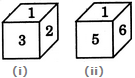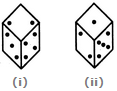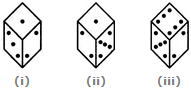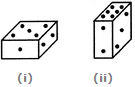# Non Verbal Reasoning - Cubes and Dice

### Exercise :: Cubes and Dice - Section 2

6.

Two positions of a dice are shown. When 4 is at the bottom, what number will be on the top?A. 1 B. 2 C. 5 D. 6

Answer: Option A

Explanation:

From figures (i) and (ii), we conclude that 2, 3, 5 and 6 he adjacent to 1. Therefore, 4 lies opposite 1. Hence, when 4 is at the bottom, then1 must be on the top.

7.

A dice is rolled twice and the two positions are shown in the figure below. What is the number of dots at the bottom face when the dice is in position (i)?A. 1 B. 5 C. 6 D. Cannot be determined

Answer: Option C

Explanation:

From figures (i) and (ii) we conclude that 3, 4, 1 and 5 dots appear adjacent to 2 dots. Therefore, 6 dots must appear opposite 2 dots. Since, there are 2 dots on the top face when the dice is in position (i), therefore, the number of dots at the bottom face must be 6.

8.

Below are depicted the three different positions of a dice. Find the number of dots on the face opposite to the face with one dot.A. 2 B. 3 C. 4 D. 6

Answer: Option D

Explanation:

From figures (i), (ii) and (iii), we conclude that 1, 3, 5 and 6 dots appear adjacent to the face with 2 dots. Therefore, 4 dots will appear opposite to 2 dots. Now, from figures (i) and (ii), we conclude that 2, 3 and 5 dots appear adjacent to 1 dot Therefore, either 4 or 6 dots will appear opposite to 1 dot. But since, 4 dots appear opposite to 2 dots it follows that 6 dots will appear opposite 1 dots.

9.

Two positions of a parallelepiped are shown below. When the number 3 will be on the top side, then which number will be at the bottom?A. 1 B. 4 C. 5 D. 6

Answer: Option C

Explanation:

The number 2 is common to both the figures. We assume the parallelepiped in fig. (ii) to be rotated so that 2 appears at the same position as in fig. (i) i.e. on the RHS face and the numbers 6 and 3 move to the faces hidden behind the numbers 1 and 5 respectively [in fig. (i)]. Then, the combined figure will have 1 opposite 6 and 5 opposite 3. Thus, when 3 will be on the top, then 5 will appear at the bottom.

10.

A dice is numbered from 1 to 6 in different ways.

If 1 is adjacent to 2, 3 and 5, then which of the following statements is necessarily true?

 A. 4 is adjacent to 6 B. 2 is adjacent to 5 C. 1 is adjacent to 6 D. 1 is adjacent to 4

Answer: Option A

Explanation:

If 1 is adjacent to 2, 3 and 5, then either 4 or 6 lies opposite to 1. So, the numbers 4 and 6 cannot lie opposite to each other. Hence, 4 necessarily lies adjacent to 6.

#### Current Affairs 2021

Interview Questions and Answers